Chapter 8: Exercises

1. (***) Extension to lcs.m: Write an m-file function myLcs.m which can return multiple LCS strings of the same length. The usage is
[lcsLength, lcsPath, lcsStr, lcsTable] = lcs(str1, str2, showOpt);
where "lcsPath" and "lcsStr" are cell arrays for returning multiple LCS results. The program should be able to
1. Return the right results.
2. Draw the right backtracking paths (including local and global ones) when showPlot is 1. (You need to modify the plotting function for lcs.m.)

Hint: lcs.m is available in the Machine Learning Toolbox.

2. (***) MATLAB function for type-2 DTW: Write an m-file function dtw2m.m that is equivalent to its mex version (dtw2mex.mex*) in the Machine Learning toolbox. The usage is
[minDistance, dtwPath, dtwTable] = dtw2m(vec1, vec2);
You can use the following example as a script to test your program:

Example 1: dtw2test01.mcurrDir=pwd; cd([mltRoot, '/private']); vec1=[0 1 2 3 3 2 1 0 0 1 2 1 0]; vec2=[0 0 1 2 3 2 1 0 0 0 1 3 2 0]; [minDist1, dtwPath1, dtwTable1] = dtw2m(vec1, vec2); [minDist2, dtwPath2, dtwTable2] = dtw2mex(vec1, vec2); fprintf('minDist1=%g, minDist2=%g, error=%g\n', minDist1, minDist2, abs(minDist1-minDist2)); dtwPathPlot(vec1, vec2, {dtwPath1, dtwPath2}); cd(currDir);minDist1=3, minDist2=3, error=0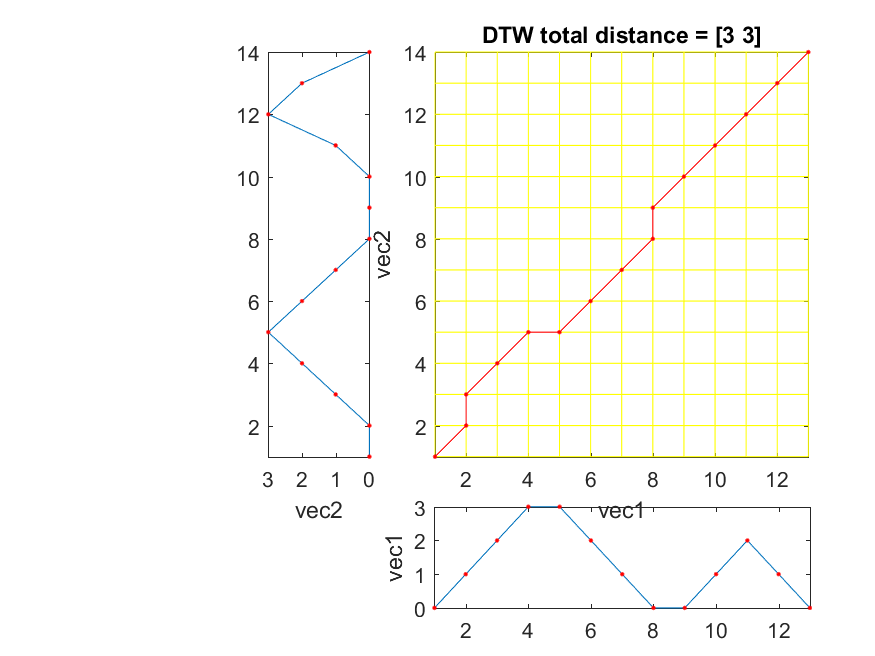For a more extensive test with timing measurement, try the following example:

Example 2: dtw2test02.m% Compare the speed/distance difference between various versions of type-1 DTW currDir=pwd; cd([mltRoot, '/private']); % ====== Output test beginCorner=1; endCorner=1; testNum=100; fprintf('%d runs of output tests:\n', testNum); for i=1:testNum vec1=randn(1, 25); vec2=randn(1, 30); [minDist1, dtwPath1, dtwTable1] = dtw2m(vec1, vec2, beginCorner, endCorner); [minDist2, dtwPath2, dtwTable2] = dtw2mex(vec1, vec2, beginCorner, endCorner); if ~isequal(minDist1, minDist2) | ~isequal(dtwPath1, dtwPath2) | ~isequal(dtwTable1, dtwTable2) figure('name', 'dtw2m()'); dtwPathPlot(vec1, vec2, dtwPath1); figure('name', 'dtw1mex()'); dtwPathPlot(vec1, vec2, dtwPath2); fprintf('Press any key to continue...\n'); pause end end fprintf('\tDone!\n'); % ====== Speed test testNum=200; mat1=randn(testNum, 25); mat2=randn(testNum, 30); fprintf('%d runs of timing tests:\n', testNum); tic for i=1:testNum, dtw2m(mat1(i,:), mat2(i,:)); end time1=toc; tic for i=1:testNum, dtw2mex(mat1(i,:), mat2(i,:)); end time2=toc; fprintf('\ttime1=%g, time2=%g, ratio=time1/time2=%g\n', time1, time2, time1/time2); cd(currDir);100 runs of output tests: Done! 200 runs of timing tests: time1=0.0138575, time2=0.00274573, ratio=time1/time2=5.04693

For simplicity, you should only consider the following situations:
• You only need to consider the case of "anchored beginning and anchored end".
• You need to add the global constraints where the feasible region is a parallelogram.
• You can assume the inputs are row vectors.
The behavior of dtw2mex.mex* should be exactly the same as those described in the text. If you find any discrepancies, feel free to let me know.
3. (***) Implementation of a speaker-dependent voice-command system using DTW: In this exercise, you are going to use MATLAB to implement a speaker-dependent voice-command system. Basically, the user can record a 3-second utterance and the system will identify the most likely command via DTW. To create such a system, we have two stage for registration and recognition, respectively. During the registration stage, we need to prepare a database where the user can register their utterances for recognition. This involves the following steps:
1. Prepare a text file "command.txt" manually. The file should contain at least 10 commands. An English example follows: one two three five ... You can also use the following Chinese example: 牛肉麵 珍珠奶茶 貢丸湯 韭菜盒子 肉粽 ... (In fact, you can use any language you like to prepare the text file.)
2. Write a script for the users to record these commands twice. To increase the variability, you should record each command once in a run, for two runs. (You can use "textread" command to read the text file.)
3. After each recording, you should perform endpoint detection and feature extraction, and store all the information in a structure array recording of size 2*n, where n is the number of voice commands in "command.txt". The structure array recording contains the following fields:
• recording(i).text: Text for the i-th recording
• recording(i).mfcc: MFCC for the i-th recording
4. Save the variable recording to recording.mat.
During the recognition stage, the user can hit any key to start 3-second recording, and the system will demonstrate the top-10 results on the screen according to the DTW distance. The recognition stage involved the following steps:
2. Obtain the user's 3-sec utterance.
3. Perform endpoint detection and feature extraction.
4. Compare the MFCC with that of each recording in the array recording using DTW.
5. Rank the distance.
6. List the top-10 result (by showing the texts) according to the distance.
7. Go back to step ii unless ctrl-c is hit.
Some hints follow:
• All recordings are of the format: 16KHz, 16Bits, mono.
• The system should allow the user to do repeated recordings until ctrl-C is hit.
• You can use epdByVolHod.m for endpoint detection. After each recording, the result of endpoint detection should be displayed on the screen immediately to facilitate debugging. (It is in the SAP Toolbox.)
• You can use wave2mfcc.m for feature extraction. (It is in the SAP Toolbox.)
• You can use either dtw.m (in the Machine Learning toolbox) for DTW.
When you demo your system to TA, make sure you can have at least 3 utterances to have the correct answer in top-1 results.

4. (***) Programming contest: Use DTW for speaker-dependent speech recognition: Please follow this link to have more descriptions.

5. (***) Programming contest: Dice sum game: In this exercise, you need to design a strategy for playing the dice sum game. The game is very simple. You need to toss a dice 8 times and place the dice values into 4 2-digit numbers. If the sum of these 4 numbers are less than or equal to 150, your score is the sum. Otherwise, your score is zero. Note that you need to place the dice value right after each toss. The sample code is available here. Please run goGameEval.m to see how the TA perform the evaluation of your strategy. You need to submit the following files:
• myStrategy.m (If you need to load some parameters, please also submit the mat files.)
• method.txt: A one-page summary stating how you tackle the problem.
TA will run your program and post the results regularly to the leaderboard.

Hint
It is possible to use DP to find the optimum strategy of the game. You can come up with your own strategy to optimize the overall performance.

6. (***) Plateau passing via DP: A plateau (高原) is represented by a m-by-n matrix $P$. A path through the plateau can be represented by a function $f(i), i=1,2,\dots,n$, which indicates the row index at column $i$. More precisely, the path can be expressed by a set of $n$ index-pairs into matrix $P$: $$\{\left(f(i), i\right)|i=1,2,\dots,n, 1 \leq f(i)\leq m\}.$$ Given the above path, the objective function to be maximized can be defined as: $$obj(\alpha)=\sum_{i=1}^n P(f(i), i) - \sum_{i=1}^{n-1}\alpha |f(i)-f(i+1)|$$ where $\alpha$ is a positive penalty coefficient. In other words, the objective function involves two terms:
1. The first term indicates we need to have a path as high as possible.
2. The second term indicates we need to have a path as straight as possible.
The above two criteria are mixed by the penalty coefficient $\alpha$:
1. If $\alpha=0$, then the path should be located at the max of each column of $P$.
2. If $\alpha=\infty$, then the path should be a horizontal straight line obtained from the maximum of row sum of $P$.
You mission is to write a function that can maximize the objective function by finding the maximizing path for given $P$ and $\alpha$, based on the principal of DP. The I/O format of the function is shown next:
[optimValue, optimPath]=myPlateauPass(P, alpha);
where
• optimValue: The max. value of the objective function
• optimPath: A row vector indicating the maximizing path of $f(i), i=1,2,\dots,n$.
Here are some test examples.
1. This example shows the case when $\alpha$ is 0, with a small-size $P$.

Example 3: myPlateauPass01.mP=[3 6 7 5;8 5 2 9;8 3 9 7]; alpha=0; [optimValue, optimPath]=myPlateauPass(P, alpha); fprintf('optimValue=%g\n', optimValue); fprintf('optimPath=%s\n', mat2str(optimPath)); % Plotting [m, n]=size(P); imagesc(1:n, 1:m, P); axis image; shading flat; colorbar title('Plateau'); for i=1:n line(i, optimPath(i), 'color', 'k', 'marker', '.'); endoptimValue=32 optimPath=[2 1 3 2]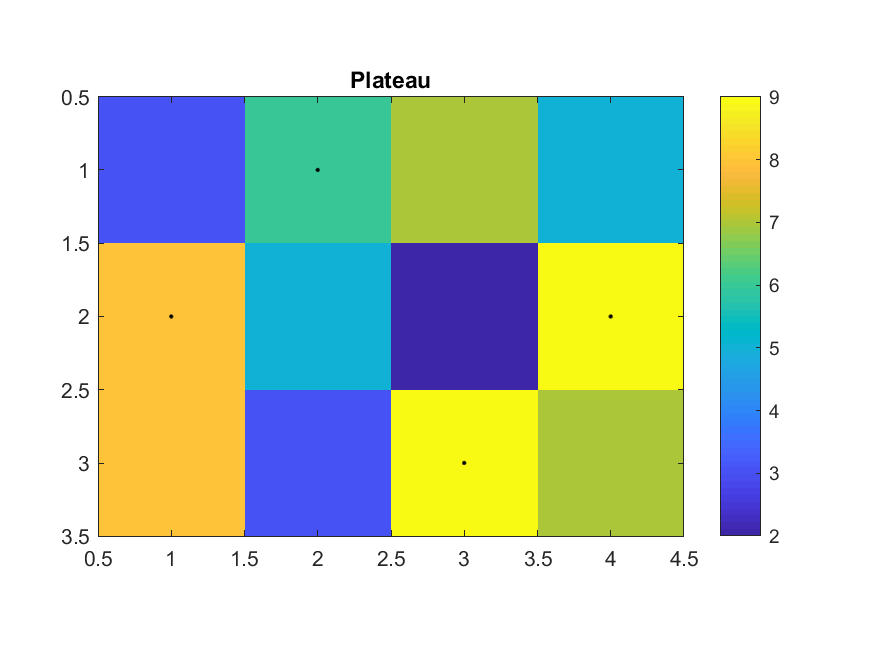2. This example shows the case when $\alpha$ is $\infty$, with a small-size $P$.

Example 4: myPlateauPass02.mP=[3 6 7 5;8 5 2 9;8 3 9 7]; alpha=inf; [optimValue, optimPath]=myPlateauPass(P, alpha); fprintf('optimValue=%g\n', optimValue); fprintf('optimPath=%s\n', mat2str(optimPath)); % Plotting [m, n]=size(P); imagesc(1:n, 1:m, P); axis image; shading flat; colorbar title('Plateau'); for i=1:n line(i, optimPath(i), 'color', 'k', 'marker', '.'); endoptimValue=27 optimPath=[3 3 3 3]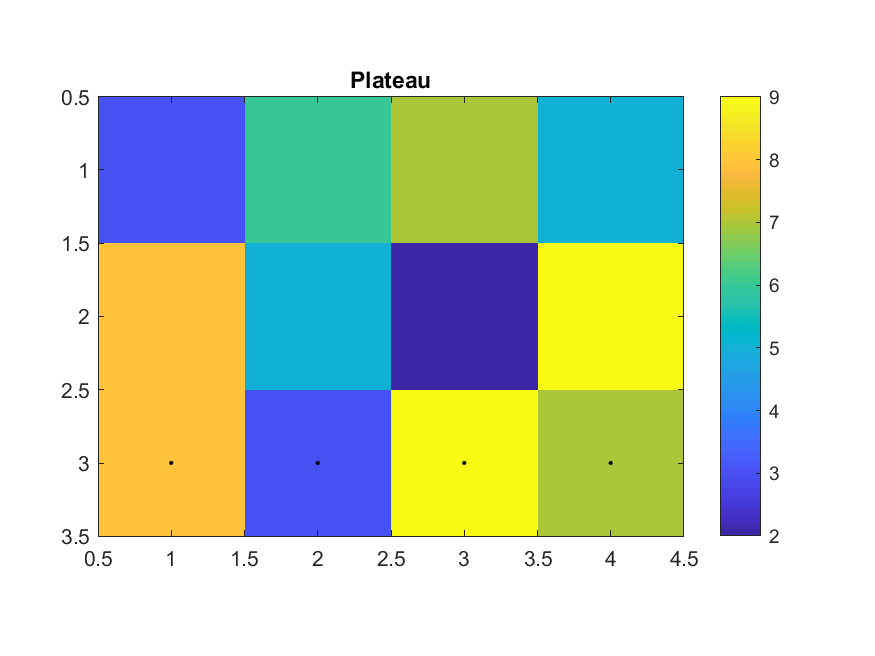3. This example shows the case when $\alpha$ is 0, with a median-size $P$.

Example 5: myPlateauPass03.mP=[3 6 7 6 2 5 7 0 5 9 5 7 2 7 5 3 1 3 5 7;8 6 6 5 6 0 0 8 8 7 9 1 9 0 2 7 9 5 3 5;8 9 9 7 8 10 9 1 4 2 7 9 4 7 9 6 3 4 1 8;3 3 9 3 1 1 7 6 4 5 2 9 2 4 2 5 3 9 2 5;10 7 2 9 4 2 4 10 9 1 4 6 8 4 8 6 2 10 6 8;6 1 1 9 8 6 9 4 2 10 3 4 5 6 3 4 2 2 1 1;2 2 7 5 7 10 1 7 3 4 6 4 2 0 2 9 8 8 7 10;5 9 3 5 8 5 4 9 10 4 8 4 5 5 3 7 3 3 4 5;0 4 7 9 6 5 7 6 6 5 6 5 7 8 7 4 5 2 6 8;0 3 9 6 3 2 3 1 9 9 7 2 6 10 5 8 3 1 1 4]; alpha=0; [optimValue, optimPath]=myPlateauPass(P, alpha); fprintf('optimValue=%g\n', optimValue); fprintf('optimPath=%s\n', mat2str(optimPath)); % Plotting [m, n]=size(P); imagesc(1:n, 1:m, P); axis image; shading flat; colorbar title('Plateau'); for i=1:n line(i, optimPath(i), 'color', 'k', 'marker', '.'); endoptimValue=185 optimPath=[5 3 3 5 3 3 3 5 8 6 2 3 2 10 3 7 2 5 7 7]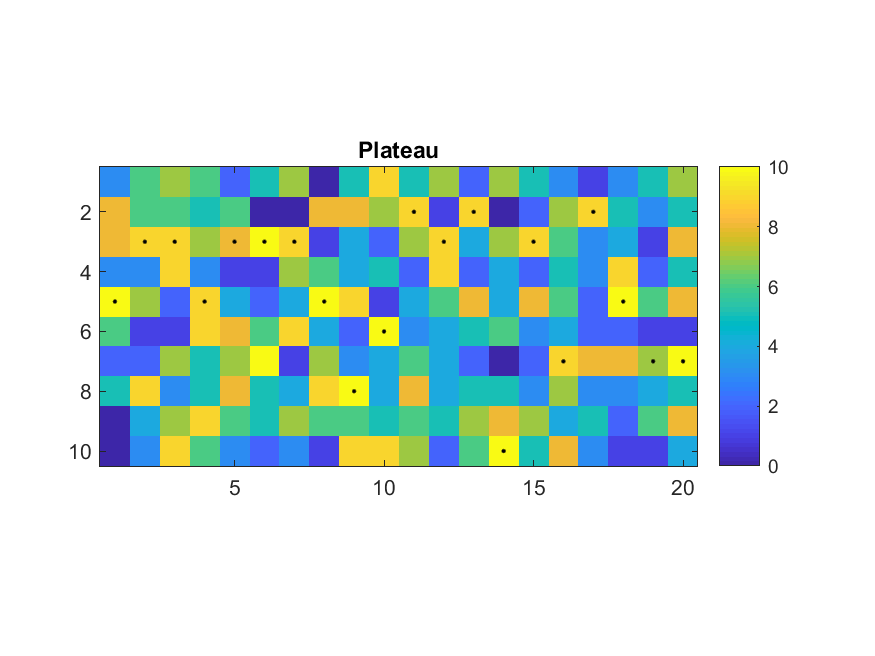4. This example shows the case when $\alpha$ is $\infty$, with a median-size $P$.

Example 6: myPlateauPass04.mP=[3 6 7 6 2 5 7 0 5 9 5 7 2 7 5 3 1 3 5 7;8 6 6 5 6 0 0 8 8 7 9 1 9 0 2 7 9 5 3 5;8 9 9 7 8 10 9 1 4 2 7 9 4 7 9 6 3 4 1 8;3 3 9 3 1 1 7 6 4 5 2 9 2 4 2 5 3 9 2 5;10 7 2 9 4 2 4 10 9 1 4 6 8 4 8 6 2 10 6 8;6 1 1 9 8 6 9 4 2 10 3 4 5 6 3 4 2 2 1 1;2 2 7 5 7 10 1 7 3 4 6 4 2 0 2 9 8 8 7 10;5 9 3 5 8 5 4 9 10 4 8 4 5 5 3 7 3 3 4 5;0 4 7 9 6 5 7 6 6 5 6 5 7 8 7 4 5 2 6 8;0 3 9 6 3 2 3 1 9 9 7 2 6 10 5 8 3 1 1 4]; alpha=inf; [optimValue, optimPath]=myPlateauPass(P, alpha); fprintf('optimValue=%g\n', optimValue); fprintf('optimPath=%s\n', mat2str(optimPath)); % Plotting [m, n]=size(P); imagesc(1:n, 1:m, P); axis image; shading flat; colorbar title('Plateau'); for i=1:n line(i, optimPath(i), 'color', 'k', 'marker', '.'); endoptimValue=125 optimPath=[3 3 3 3 3 3 3 3 3 3 3 3 3 3 3 3 3 3 3 3]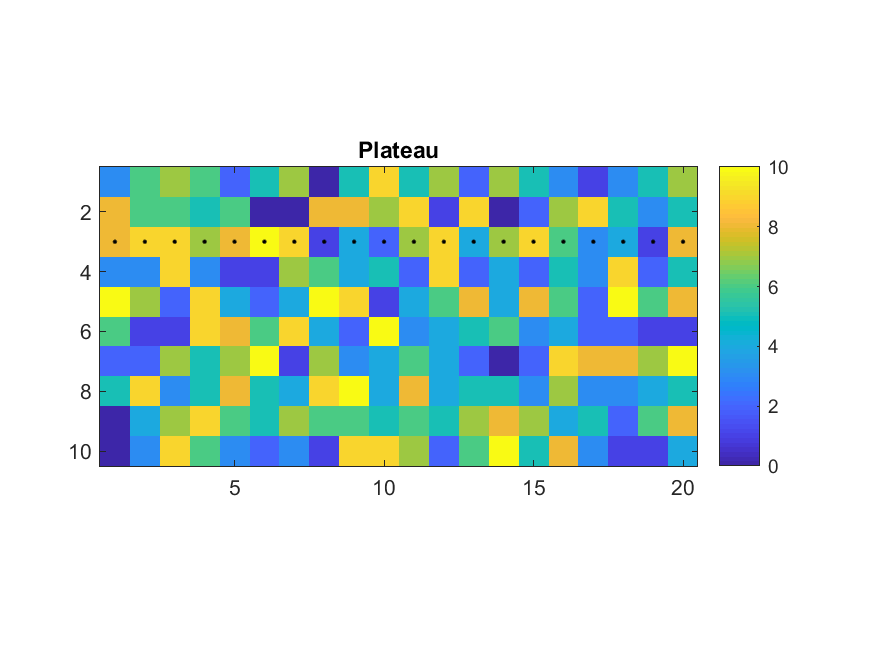5. This example shows a real case when $P$ is $320 \times 243$. It takes about 0.2 seconds on my notebook. Non-DP approaches will take much longer.

Example 7: myPlateauPass05.mload pfMat.mat pfMat(1:20, :)=0; P=pfMat; alpha=10000; tic [optimValue, optimPath]=myPlateauPass(P, alpha); fprintf('optimValue=%g\n', optimValue); fprintf('optimPath=%s\n', mat2str(optimPath)); toc % Plotting subplot(2,1,1); [m, n]=size(P); imagesc(1:n, 1:m, P); axis image; shading flat; colorbar title('Plateau'); for i=1:n line(i, optimPath(i), 'color', 'k', 'marker', '.'); end subplot(2,1,2); mesh(1:n, 1:m, P); axis ij; axis tight; colorbar; box on for i=1:n line(i, optimPath(i), P(optimPath(i), i), 'color', 'k', 'marker', '.'); end title('Opt. path over the plateau'); optimValue=2.48802e+07 optimPath=[88 88 88 88 88 88 88 88 88 88 88 88 88 88 88 88 88 89 90 91 92 94 96 97 100 103 106 111 113 116 119 121 123 123 124 126 128 128 128 128 128 128 128 128 128 128 128 128 128 128 128 128 128 128 128 128 128 128 130 132 134 136 136 136 136 136 136 136 137 139 139 139 139 139 139 139 139 139 138 137 136 135 134 133 133 133 132 131 130 130 130 130 130 129 127 118 116 116 115 115 115 115 115 115 115 115 115 116 116 116 116 116 117 117 117 119 121 123 124 124 124 124 124 124 124 124 124 121 120 119 117 117 117 117 117 117 117 117 117 107 102 102 102 102 102 102 102 102 102 102 101 101 100 100 100 100 100 99 97 96 96 96 96 96 96 96 94 91 91 91 91 91 92 95 98 100 102 105 107 108 111 112 112 112 112 112 112 112 112 112 112 112 112 112 111 111 105 105 95 94 94 94 94 96 99 102 103 107 113 118 121 127 131 137 137 137 137 137 137 137 137 137 137 137 137 137 137 137 137 137 137 137 137 137 137 137 137 137 137 137 137 137 137] Elapsed time is 0.200363 seconds.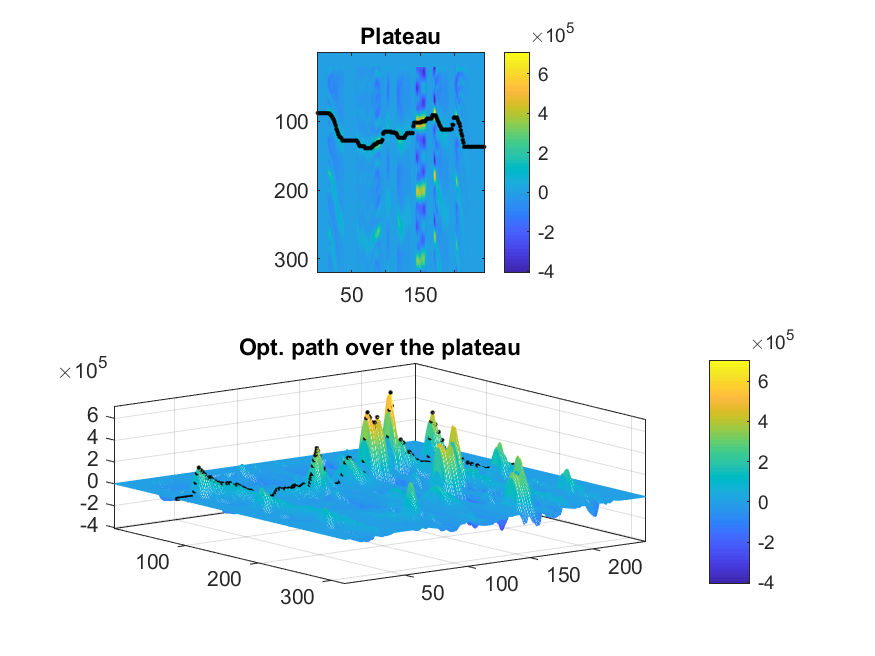Data Clustering and Pattern Recognition (資料分群與樣式辨認)